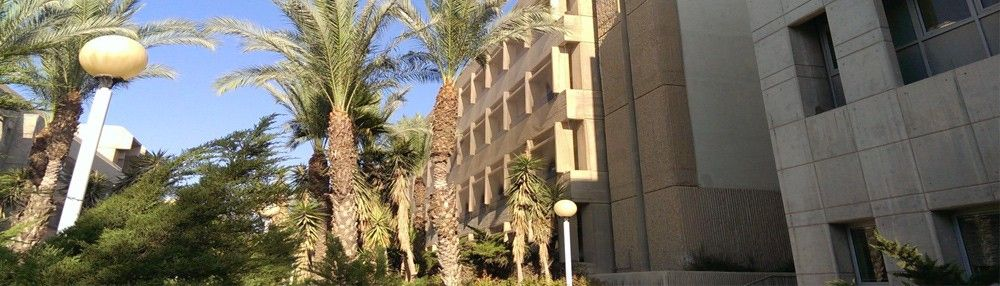•Basic methods in numerical analysis will be oerviewd in the context of various themes in Chemical-Engineering, such as mass-balances, thermodynamics, separation, flow in a channel, correlations, heat transfer and reactors. The numerical implementation in Matlab and Polymath will include code-writing and algorythmic elements.

Syllabus

I.     Mathematical modeling.
II.    Matrix operation.
III.   Basic algorithms.
IV.   Logic and boolean operations.
V.    Set of linear equations.
VI.   Polynomial approxiamtions (linearization, interpolation-extrapolation).
VII.  Solution of nonlinear equation.
VIII. Optimization
IX.    Regression (linear and nonlinear).

X.     Solution of an ordinary differential equation.

Bibliography

1.      M.B Cutlip & M .Shacham, "Problem Solving in Chemical and Biochemical Engineering with            POLYMATH, Excel and MATLAB", 2nd Ed., Prentice Hall PTR, 2008.

2.      J.B. Riggs, “An Introduction to Numerical Methods for Chemical Engineers”, 2nd Ed., Texas            Tech University Press, 1994.

3.      S.C. Chapra & R.P. Canale, “Numerical Methods for Engineers”, 6th Ed., McGraw Hill, 2010.

2all - Web Design